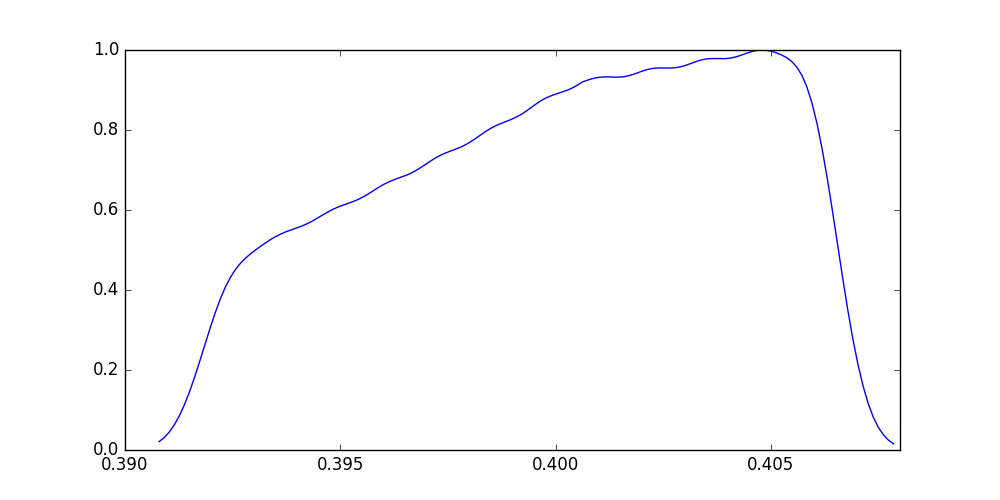# Usage¶

Get the spectral responses for Sentinel-3A OLCI (and turn on debugging to see more info on what is being done behind the curtan):

```>>> from pyspectral.rsr_reader import RelativeSpectralResponse
>>> from pyspectral.utils import debug_on
>>> debug_on()
>>> olci = RelativeSpectralResponse('Sentinel-3A', 'olci')
```

You will see that if you haven’t run this kind of code before, pyspectral will download the spectral responses for all satellites supported from Zenodo.

Now, you can work with the data as you wish, make some simple plot for instance:

```>>> [str(b) for b in olci.band_names]
['Oa01', 'Oa02', 'Oa03', 'Oa04', 'Oa05', 'Oa06', 'Oa07', 'Oa08', 'Oa09', 'Oa10', 'Oa11', 'Oa12', 'Oa13', 'Oa14', 'Oa15', 'Oa16', 'Oa17', 'Oa18', 'Oa19', 'Oa20', 'Oa21']
>>> print("Central wavelength = {wvl:7.6f}".format(wvl=olci.rsr['Oa01']['det-1']['central_wavelength']))
Central wavelength = 0.400303
>>> import matplotlib.pyplot as plt
>>> dummy = plt.figure(figsize=(10, 5))
>>> import numpy as np
>>> rsr = olci.rsr['Oa01']['det-1']['response']
>>> resp = np.where(rsr < 0.015, np.nan, rsr)
>>> wl_ = olci.rsr['Oa01']['det-1']['wavelength']
>>> wvl = np.where(np.isnan(resp), np.nan, wl_)
>>> dummy = plt.plot(wvl, resp)
>>> plt.show()
```A simple use case:

```>>> from pyspectral.rsr_reader import RelativeSpectralResponse
>>> modis = RelativeSpectralResponse('EOS-Aqua', 'modis')
>>> sflux = solar_irr.inband_solarflux(modis.rsr['20'])
>>> print("Solar flux over Band: {sflux}".format(sflux=round(sflux, 6)))
Solar flux over Band: 2.002928
```

And, here is how to derive the solar reflectance (removing the thermal part) of the Aqua MODIS 3.7 micron band:

```>>> from pyspectral.near_infrared_reflectance import Calculator
>>> import numpy as np
>>> sunz = np.array([80.])
>>> tb3 = np.array([290.0])
>>> tb4 = np.array([282.0])
>>> refl37 = Calculator('EOS-Aqua', 'modis', '20', detector='det-1', solar_flux=2.0029281634299041)
>>> print("Reflectance = {r:7.6f}".format(r=np.ma.round(refl37.reflectance_from_tbs(sunz, tb3, tb4), 6)))
Reflectance = 0.251249
```

And, here is how to derive the atmospheric (Rayleigh scattering by atmospheric molecules and atoms as well as Mie scattering and absorption of aerosols) contribution in a short wave band:

```>>> from pyspectral import rayleigh
>>> rcor = rayleigh.Rayleigh('GOES-16', 'abi')
>>> import numpy as np
>>> sunz = np.array([[50., 60.], [51., 61.]])
>>> satz = np.array([[40., 50.], [41., 51.]])
>>> azidiff = np.array([[160, 160], [160, 160]])
>>> redband =  np.array([[0.1, 0.15], [0.11, 0.16]])
>>> refl = rcor.get_reflectance(sunz, satz, azidiff, 'ch2', redband)
>>> print([np.round(r, 6) for r in refl.ravel()])
[3.254637, 6.488645, 3.434351, 7.121556]
```